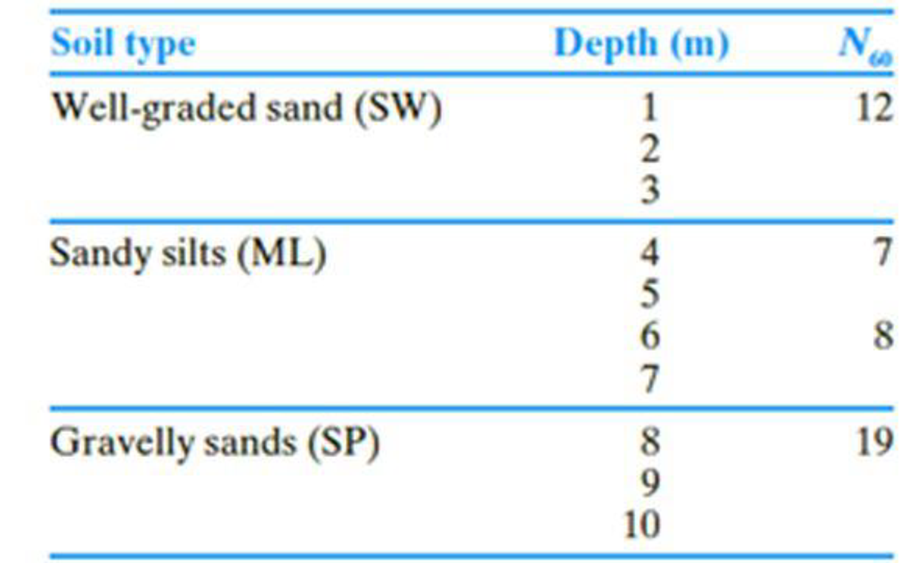Chapter 16, Problem 16.1CTPPrinciples of Geotechnical Enginee...

9th Edition
Braja M. Das + 1 other
ISBN: 9781305970939

Solutions

Chapter
SectionPrinciples of Geotechnical Enginee...

9th Edition
Braja M. Das + 1 other
ISBN: 9781305970939
Textbook Problem

The following table shows the boring log at a site where a multi-story shopping center would be constructed. Soil classification and the standard penetration number, N60, are provided in the boring log. All columns of the building are supported by square footings which must be placed at a depth of 1.5 m. Additionally, the settlement (elastic) of each footing must be restricted to 20 mm. Since the column loads at different location can vary, a design chart is helpful for quick estimation of footing size required to support a given load. a. Prepare a chart by plotting the variation of maximum allowable column loads with footing sizes, B = 1 m, 1.5 m, 2 m, and 3 m. Use a factor of safety of 3. b. If the gross column load from the structure is 250 kN, how would you use this chart to select a footing size? c. What would be the design footing size for the column in Part (b) if you use Terzaghi’s bearing capacity equation? For the well graded sand, assume that ϕ′ = 33°. Use Fs = 3. d. Compare and discuss the differences in footing sizes obtained in Parts b and c.(a)

To determine

Plot the variation of maximum allowable column loads with size of footings to prepare a chart.

Explanation

Given information:

The location of depth of footing Df is 1.5 m.

The given size of the footing B is 1 m, 1.5 m, 2 m, and 3 m.

The settlement of each footing Se is 20 mm.

The given factor of safety Fs is 3.

Calculation:

For B value is 1 m:

Determine the depth factor using the relation.

Fd=1+0.33DfB

Substitute 1.5 m for Df and 1.0 m for B.

Fd=1+0.33×1.51.0=1.495

The Fd value should be less than 1.33. The calculated Fd(1.495)>1.33. Take Fd as 1.33.

The field standard penetration number N60 should be averaged up to a distance of 2 m (2×1) below the foundation.

Determine the depth of foundation for the field standard penetration number gets averaged.

depth=Df+2B

Substitute 1.5 m for Df and 1 m for B.

depth=1.5+2(1)=3.5m

Determine the averaged N60 value using the relation.

N60=(N60)SW+(N60)ML2

Here, (N60)SW is the field standard penetration number of well graded sand and (N60)ML is the field standard penetration number of sandy silts.

Substitute 12 for (N60)SW and 7 for (N60)ML.

N60=12+72=9.510

Determine the net allowable bearing capacity of the soil (qnet) using the relation.

qnet=N600.05Fd[Se25]

Substitute 10 for N60, 1.33 for Fd, and 20 mm for Se.

qnet=100.05×1.33=212.8kN/m2

Determine the maximum allowable column load Qall-net using the relation.

Qall-net=qnet×B2Fs

Substitute 212.8kN/m2 for qnet, 1 m for B, and 3 for Fs.

Qall-net=212.8×123=71kN

For B value is 1.5 m:

Determine the depth factor using the relation.

Fd=1+0.33DfB

Substitute 1.5 m for Df and 1.5 m for B.

Fd=1+0.33×1.51.5=1.33

The field standard penetration number N60 should be averaged up to a distance of 3 m (2×1.5) below the foundation.

Determine the depth of foundation for the field standard penetration number gets averaged.

depth=Df+2B

Substitute 1.5 m for Df and 1.5 m for B.

depth=1.5+2(1.5)=4.5m

Determine the averaged N60 value for 4.5 m depth using the relation.

N60=(N60)4+(N60)62

Here, (N60)4 is the field standard penetration number for 4 m depth and (N60)6 is the field standard penetration number for 6 m depth.

Substitute 7 for (N60)4 and 8 for (N60)6.

N60=7+82=7.58

Determine the net allowable bearing capacity of the soil (qnet) using the relation.

qnet=N600.08(B+0.3B)2Fd[Se25]

Substitute 8 for N60, 1.33 for Fd, and 20 mm for Se.

qnet=80.08×(1.5+0.31.5)2×1.33=153.2kN/m2

Determine the maximum allowable column load Qall-net using the relation.

Qall-net=qnet×B2Fs

Substitute 153.2kN/m2 for qnet, 1.5 m for B, and 3 for Fs.

Qall-net=153.2×1.523=115kN

For B value is 2 m:

Determine the depth factor using the relation.

Fd=1+0

(b)

To determine

Find the footing size for the given gross column load of 250 kN.

(c)

To determine

Find the design column load for the footing size of 2.25 m using the Terzaghi’s bearing capacity equation.

(d)

To determine

Compare and discuss the differences in footing sizes obtained in parts (b) and (c).

Still sussing out bartleby?

Check out a sample textbook solution.

See a sample solution

The Solution to Your Study Problems

Bartleby provides explanations to thousands of textbook problems written by our experts, many with advanced degrees!

Get Started

What is sparse data? Give an example.

Database Systems: Design, Implementation, & Management

Class A fire extinguishers use ______ as a media to put out fires.

Precision Machining Technology (MindTap Course List)

Determine the resultant of the position vectors A and B.

International Edition---engineering Mechanics: Statics, 4th Edition

Convert P = 5.00 atm into Pa, bar, and psia.

Fundamentals of Chemical Engineering Thermodynamics (MindTap Course List)

Use an elastic analysis and determine the maximum load per inch of weld.

Steel Design (Activate Learning with these NEW titles from Engineering!)

What is the purpose of a docking station?

Enhanced Discovering Computers 2017 (Shelly Cashman Series) (MindTap Course List)